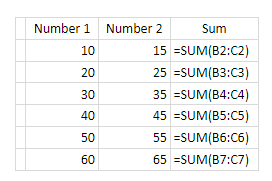# How to Display & Print Formulas in Excel & Google Sheets

### Toggle Formula View Mode

PC Shortcut: CTRL + `

Mac Shortcut: ^ + `

The grave accent (`) is on the same key as the tilde (~), at the top left of the keyboard.

See All Excel Shortcuts

This tutorial demonstrates how to display and print formulas in Excel and Google Sheets.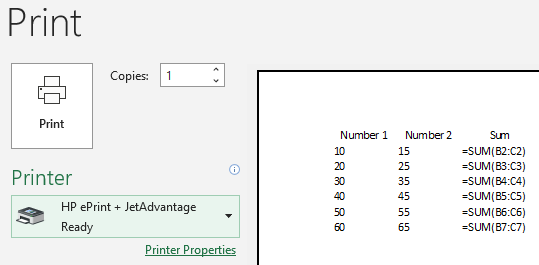## Display and Print Formulas

In Excel, it’s possible to print formulas instead of their results. You can achieve this either by turning on Show Formulas or using the FORMULATEXT Function. Say you have the SUM Function in Column D, summarizing values from Columns B and C.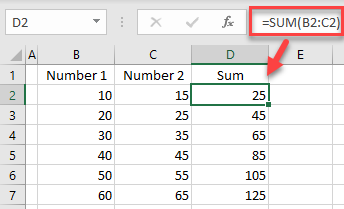1. First, to display the formulas, in the Ribbon go to Formulas > Show Formulas (or use the keyboard shortcut CTRL + `).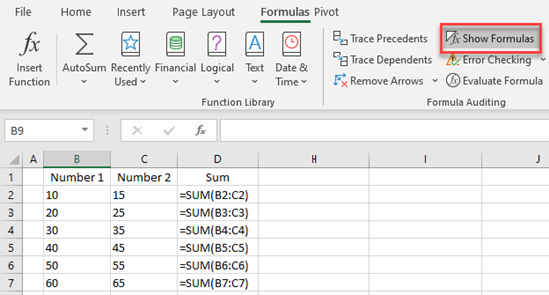1. Now all formulas in the workbook are displayed as text. To print, in the Ribbon go to File > Print (or use CTRL + P).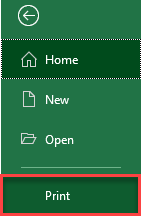As a result, all formulas print as text, as you can see in Print Preview mode.## Display and Print Formulas in Google Sheets

1. To display formulas, in the Menu, go to View > Show > Formulas (or use CTRL + `).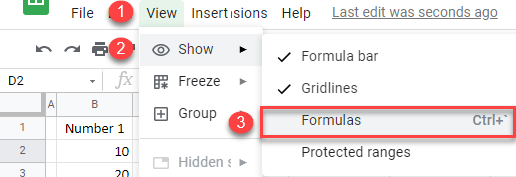1. In the Menu, go to File > Print (or use CTRL + P).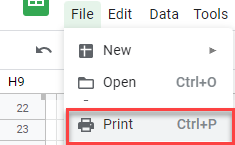In Print Preview, you can see that, instead of results, there are formulas displayed as text.